### iOS 之UI進階-CALayer創建時鐘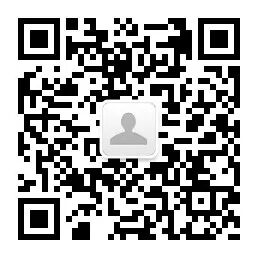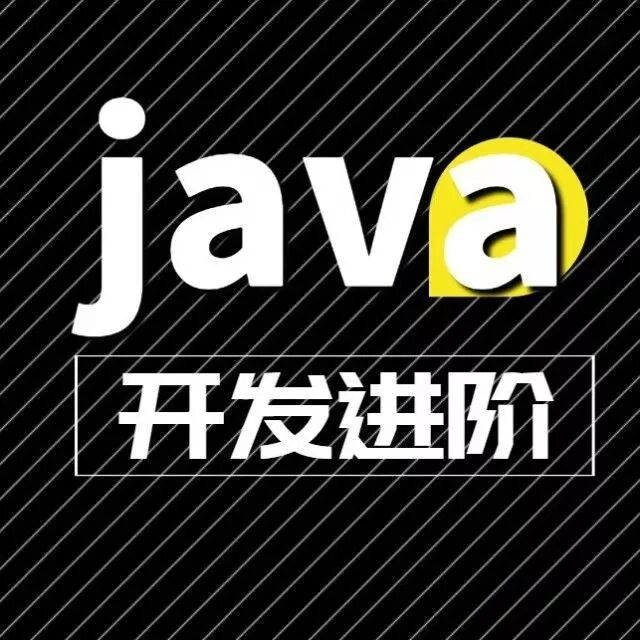Java開發進階Linux編程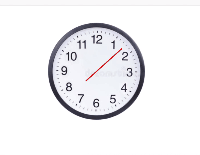## `CALayer`和`UIView`的區別

### 首先創建錶盤

`` //創建一個錶盤    CALayer* clock = [[CALayer alloc]init];    clock.bounds = CGRectMake(0, 0, 200, 200);    clock.position = CGPointMake(200, 200);    clock.contents = (__bridge id)[UIImage imageNamed:@"clock"].CGImage;    clock.cornerRadius = 100;    clock.masksToBounds = YES;``

##### 具體可以看下圖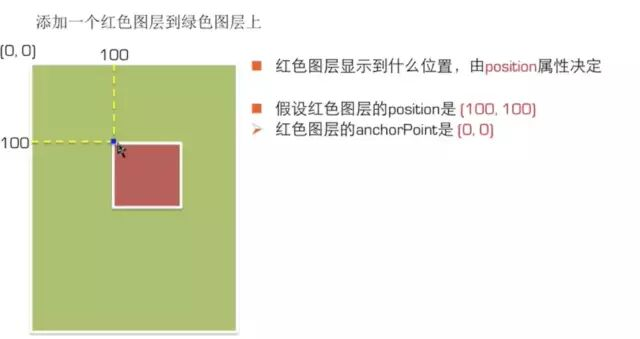### 創建秒針

`  `  //創建秒針    CALayer* second = [[CALayer alloc]init];    second.bounds = CGRectMake(0, 0, 2, 100);    second.position = clock.position;    second.backgroundColor = UIColor.redColor.CGColor;    //錨點（定位點）取值範圍是（0～1）    second.anchorPoint = CGPointMake(0.5, 0.7);``

### 時鐘刷新

##### 創建計時器

``CADisplayLink* link = [CADisplayLink displayLinkWithTarget:self selector:@selector(timeChanged)];    [link addToRunLoop:[NSRunLoop mainRunLoop] forMode:NSDefaultRunLoopMode];``
##### 刷新方法

``-(void)timeChanged{    //計算一秒鐘旋轉的角度    CGFloat angle = 2 * M_PI / 60;        NSDate* date = [NSDate date];    NSCalendar* calendar = [NSCalendar currentCalendar];    CGFloat time = [calendar component:NSCalendarUnitSecond fromDate:date];    self.second.affineTransform = CGAffineTransformMakeRotation(time*angle);}``

## `CGAffineTransformMake`和`CGAffineTransform`的區別

``CGAffineTransformMakeRotation(angle) //在最開始的位置上進行旋轉CGAffineTransformRotate(self.second.affineTransform, angle);//在原來的基礎上旋轉``

推薦↓↓↓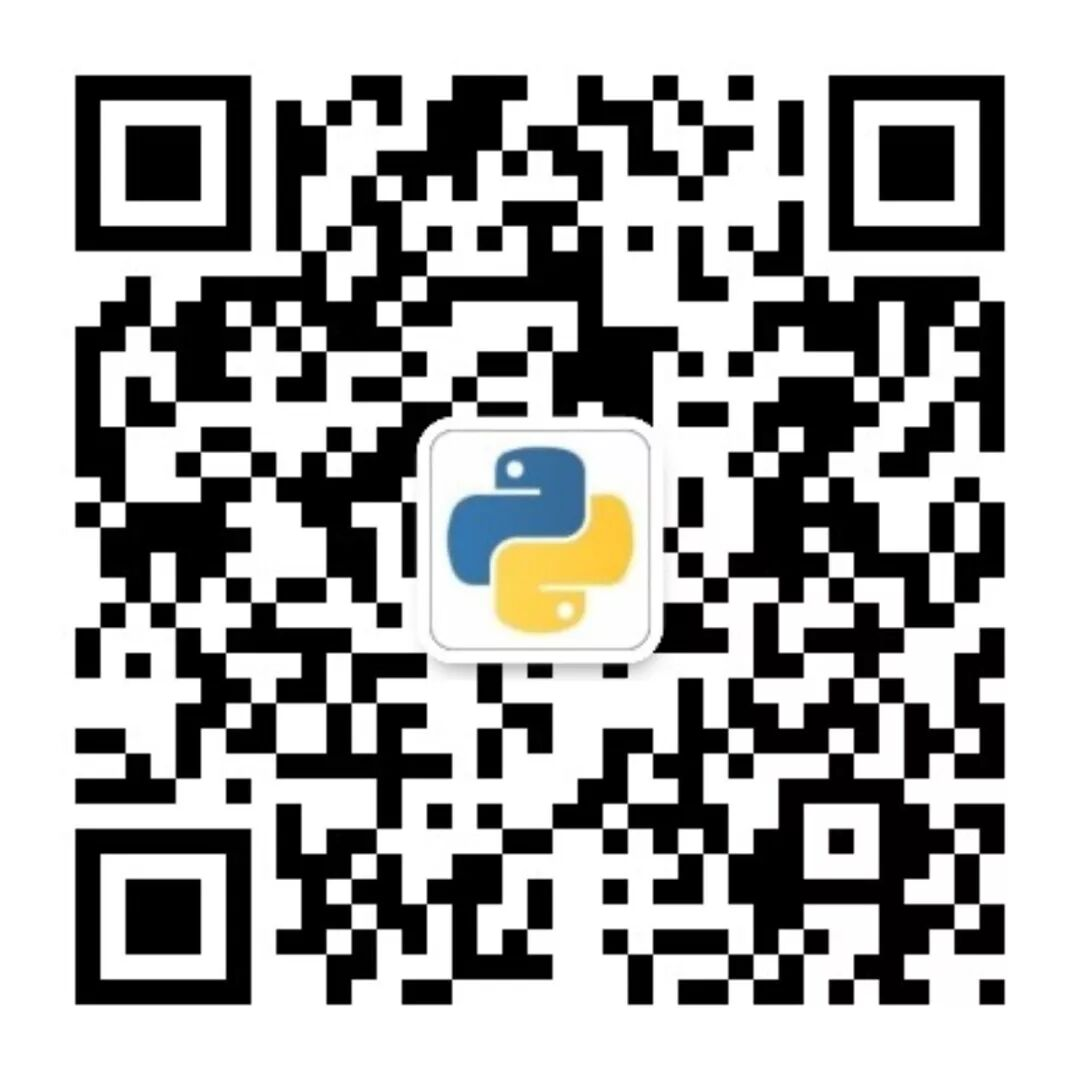👉16個技術公眾號】都在這裏！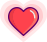https://hk.wxwenku.com/d/201135935